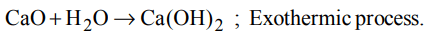## Thermodynamics Questions and Answers Part-6

1. $S+\frac{3}{2}O_{2}\rightarrow SO_{3}+2x kcal$
$SO_{2}+\frac{1}{2}O_{2}\rightarrow SO_{3}+y kcal$
Find out the heat of formation of $SO_{2}$
a) (y -2x)
b) (2x + y)
c) (x + y)
d) 2x/y

Explanation:2. The enthalpy of neutralisation of a weak acid by a strong base is
a) – 57.32 kJ
b) +57.32 kJ
c) equal to -57.32kJ + enthalpy of ionization of week acid
d) more than –57.32 kJ

Explanation: It is always = – 57.32 + ionisation of weak acid

3. The enthalpy of formation for $C_{2}H_{4}\left(g\right),CO_{2}\left(g\right) and H_{2}O\left(l\right)$       at 25º C and 1 atm pressure are 52, –394 and $– 286 kJmol^{-1}$  respectively. The enthalpy of combustion of$C_{2}H_{4}\left(g\right)$   will be
a) +1412 kJ $mol^{-1}$
b) -1412 kJ $mol^{-1}$
c) 141.2 kJ $mol^{-1}$
d) -141.2 kJ $mol^{-1}$

Explanation:4. When water is added to quicklime, the reaction is
a) explosive
b) endothermic
c) exothermic
d) photochemical

Explanation:5. Mark the correct statement
a) For a chemical reaction to be feasible, $\triangle G$  should be 0
b) Entropy is a measure of order in a system
c) For a chemical reaction to be feasible, $\triangle G$   should be positive
d) The total energy of an isolated system is constant

Explanation: Energy of isolated system is constant

6. One mole of an ideal gas is allowed to expand freely and adiabatically into vaccum until its volume has doubled. The expression which is not true concerning statement is
a) $\triangle H=0$
b) $\triangle S=0$
c) $\triangle E=0$
d) W = 0

Explanation: W = 0

7. Which of the following statements is true ?
a)$\triangle G$   may be lesser or greater or equal to $\triangle H$
b) $\triangle G$   is always proportional to $\triangle H$
c) $\triangle G$   is always greater than $\triangle H$
d) $\triangle G$   is always less than $\triangle H$

Explanation: Depending upon the value of T$\triangle$S, $\triangle$G can be lesser, equal or greater to $\triangle$H

8. Compounds with high heat of formation are less stable because
a) it is difficult to synthesize them
b) energy rich state leads to instability
c) high temperature is required to synthesize them
d) molecules of such compunds are distorted

Explanation: The more the energy, the lesser is the stability

9. The heat of combustion of $CH_{4}\left(g\right)$  , C (graphite), $H_{2}\left(g\right)$   are – 20 kcal, –40 kcal, –10 kcal respectively. The heat of formation of methane is
a) –40 kcal
b) + 40 kcal
c) –80.0 kcal
d) + 80 kcal10. $H_{2}+Cl_{2}\rightarrow 2HCl,\triangle H=- 194 kJ$        In this reaction, heat of formation of HCl in kJ is